blog

# Ultimate Guide To The Synthetic Short Stock Strategy

#### Options Trading 101 - The Ultimate Beginners Guide To Options

As Seen On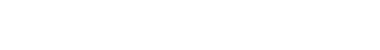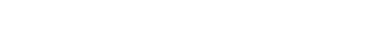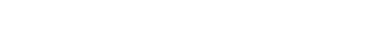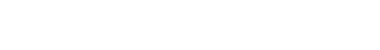by Gavin in Blog## Introduction

A synthetic short stock is a means of recreating the payoff profile of a short stock using options.

It is the sister trade to the synthetic long stock strategy.

It is a combination of a short call and long put on the same underlying stock with identical strike price and expiration.

Simply put, a synthetic short stock position uses options to replicate the payoff of shorting 100 shares of the stock without actually borrowing and selling them.

It would be unfair if I just state the definition without providing an understanding of the building block of synthetic positions – known as the put-call parity.

Put-call parity shows the position equivalence of a portfolio of a call and risk-free bond with a portfolio of underlying stock and a put.

It defines the relationship between call prices and put prices with identical strike price and expiration.

In mathematical terms, put-call parity states:

C + X/(1+r)^t = S + P.

Where,

C = Call

X = Risk free bond

S = Stock

P = Put

Basically, it means that the price of call option has some information about the price of the corresponding put option, and vice versa.

I could spend an entire month talking about the derivation of put-call parity formula, but essentially the parity holds because any material divergence between put and call values will correct themselves as arbitrageurs take advantage of the mispricing.

It only holds for options that are held to expiration, but we don’t have to worry about that for now. A simple understanding of the parity is enough to understand synthetic positions.

For the purposes of understanding synthetics, let’s assume interest rates are zero and the stock doesn’t pay a dividend.

Ok, so now that we have that out of the way. Let’s revisit the put-call parity and understand the synthetic positions formulaically and intuitively.

Formulaically if we rearrange the parity formula from above such that we have long stock on one side and the rest on the other side.

S = C – P – X(1+r)^t

We can ignore the risk-free bond (X) because all it means is that we have the money in our account to meet the strike price at expiration. Therefore, restated it is:

S = C – P (i.e. long stock equals long call plus short put)

But remember, we want to create a short stock payoff so negate everything in the above equation and we get:

-S = P – C (i.e. short stock equals long put and short call)

Formulas are fun but let’s understand the relationship intuitively.

Remember the payoff of a short stock. It states the value of our position increases as the stock price falls below the purchase price and it decreases as stock price rises above the purchase price.

A synthetic short strategy simply asks: How can we replicate the same payoff without shorting the stock?

We can do this by using options.

If we sell a call option with a strike price of x (assume x equals purchase price of stock), the payoff will decrease as the stock price rises above x, but will flatline as the stock price falls below x.

On the other hand, if we buy a put with the same strike price of x, the payoff will increase as the stock price falls below x, but will flatline as the stock price rises above x.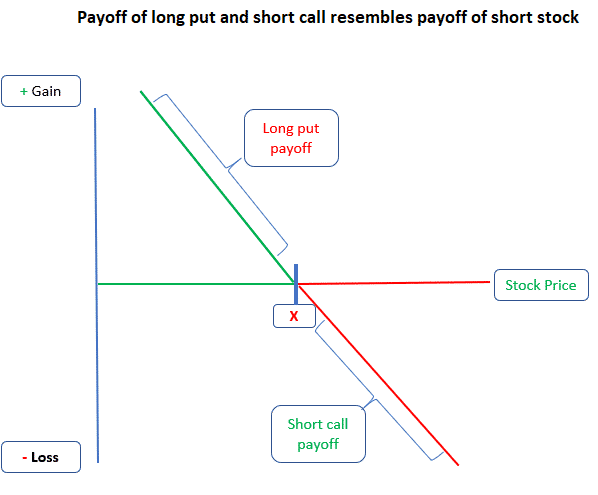This assumed there was no net debit or credit to enter the trade, and that strike price on options equaled purchase price of the stock.

We will relax these assumptions later.

Side note, when I first learned about put-call parity, I had the hardest time getting the equation straight in my head so a mnemonic I found helpful was:

Cow Boy Sings Pretty → Call + Bond = Stock + Put

Hopefully that comes in handy!

Let’s introduce an example of how the synthetic short looks and this is the example we will use for the next few sections.

An investor wants to synthetically create the same payoff as a short stock of Nikola when it is trading at \$19.95.

Unfortunately, there is no option with the same strike as the stock price so in this example, the investor selects the long put that is slightly in the money.

If on expiration, the stock is below the strike price of \$20, we can exercise our put and receive \$20.

If on expiration, the stock is above the strike price of \$20, the call will be exercised against us and we will be forced to sell the shares at \$20.

Either way, if we hold the synthetic, we will create the same payoff as if we shorted the stock.

So, why not just short the stock upfront?

Well, for a few reasons:

Shorting requires borrowing the stock to sell. The trader’s brokerage account might not allow that.

Also, unlike a synthetic short, if we borrow a dividend paying stock to short, we are responsible to pay the dividend to the lender of the stock.

Here is an example of a synthetic short stock strategy using Nikola (NKLA) stock.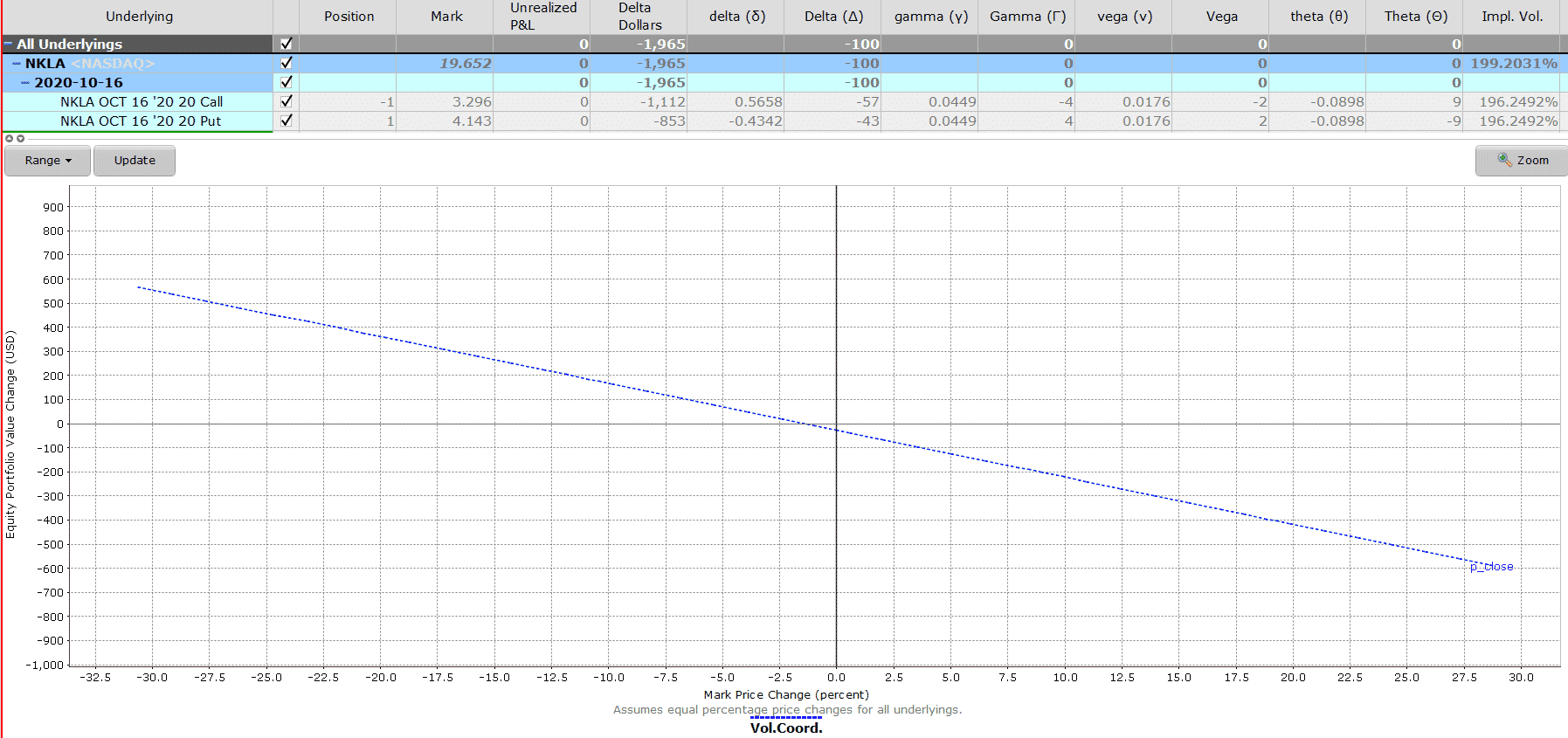## Maximum Loss

A short synthetic stock replicates the payoff of a short stock.

Therefore, the maximum loss is theoretically unlimited because stock could rise to infinity.

If the stock rises above the short call strike of \$20, the call will be assigned to us at \$20.

Our maximum loss will be stock price at expiration minus the strike on short call.

But wait, we bought a long put for \$4.14, and shorted a call for \$3.29, so we had a net debit of \$0.85.

Thus, our maximum loss needs to include the net debit paid.

## Maximum Gain

Technically the stock could fall to zero, so the most we can make is the strike price of the long put.

But remember we had a net debit of \$0.85 so maximum gain is strike price minus the net debit paid (i.e. \$19.15 = \$20 – \$0.85)

## Breakeven Price

We paid a net debit of \$0.85 to enter the synthetic position.

We will break even if the stock at expiration falls from strike of \$20 to \$19.15.

More generally, the breakeven is strike – net debit paid, or strike + net credit received.

## Payoff Diagram

The potential profit is limited to the strike price if the stock goes to zero, and the potential losses are unlimited.

The payoff diagram resembles that of a short stock position.## The Greeks

### Delta

The synthetic has two options. A long put and a short call.

Both options are usually placed at-the-money so the short call delta should be close to -0.5 and the long put delta should be close to -0.5.

The put we selected has a delta of -0.43, and the call we shorted has a delta of -0.57.

In total, the delta of our position is -1.

This is the same as the delta of the short stock of -1.

In other words, our synthetic short stock position will behave just like a short stock would.

It would gain by \$1 for every \$1 decrease in the stock price.

### Gamma

Gamma measures the rate of change in delta with respect to the change in stock price.

If gamma measures the change in delta with respect to the change in stock price, then at the money options will be highly susceptible to changes in the stock price.

Therefore, gamma of at the money calls are the highest.

The short call in our example has a gamma of -0.0449.

The long put in our example has a gamma of 0.0449.

In other words, gamma has no impact on the synthetic short stock position.

## How Volatility Impacts the Trade

Long put options are long vega trades. So, we will benefit if volatility rises after the trade has been placed.

Short call options are short vega trades. So, we will be at a disadvantage if volatility rises after the trade has been placed.

The long put has a vega of 0.0176 i.e. the value of the long put option will increase by \$0.0176 if implied volatility increases by one point.

The short call has a vega of -0.0176 i.e. the value of the short call option will decrease by \$0.0176 if implied volatility increases by one point.

In other words, the volatility on the long put fully offsets the volatility on the short call.

Therefore volatility has very little impact on the trade.

## How Theta Impacts the Trade

Theta measures how sensitive the option price is to the passage of time – i.e. how much value does the option win/lose each day as the trade approaches expiration.

We are long a put and short a call with the same strike and expiration, so loss of value in long put option will be offset by the gain in value in short call option.

The long put has theta of -0.08 and the long call has theta of 0.08.

Therefore, theta will not have a big impact on the trade.

## Risks

Just like shorting the stock, the risk of a synthetic short stock is that stock price can rise above the strike price.

When using options to replicate the payoff of a short stock position, be aware that there is a risk of early assignment.

Especially, if the underlying of the synthetic short stock is involved in a merger or buyout.

## Summary

Let’s see the results of the synthetic short position that started on

Date: Sept 24, 2020

Price: \$19.95

Sell one Oct 16 NKLA \$20 call @ \$3.296
Buy one Oct 16 NKLA \$20 put @ \$4.143

Total Debit: \$85

The investor put on the synthetic short thinking the NKLA will continue to drop.

But just as luck would have it, NKLA stopped dropping and move sideways instead.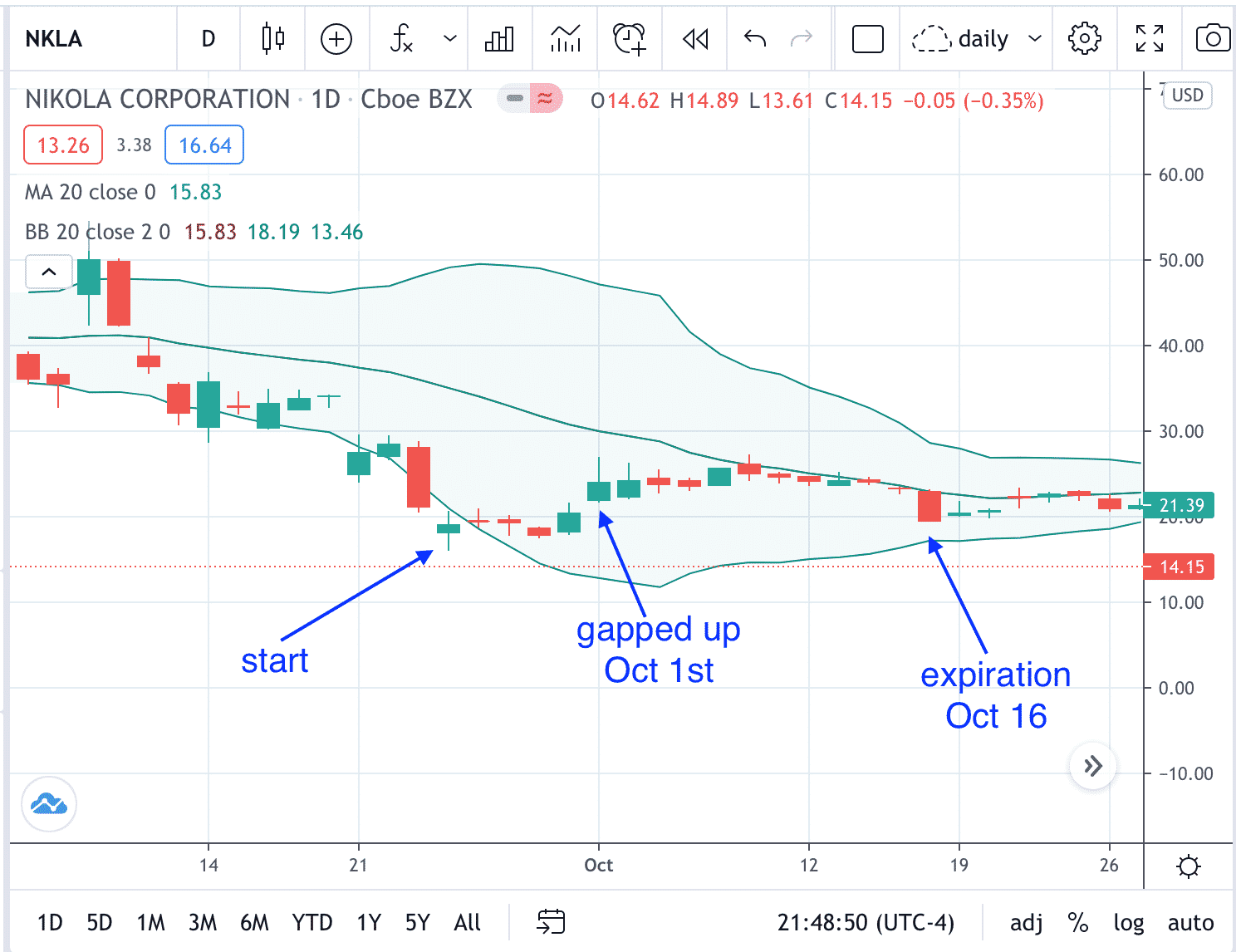On October 1st, NKLA gapped up on the open above the heads of the preceding five candles and closed at \$24.11.

This stock price action might have prompted the investor to exit. Exiting at this point results in a loss of \$470.

In comparison, a stock investor who had shorted 100 shares of NKLA would have lost \$416.

If the investor had not exited and waited till expiration when NKLA closed at \$19.55 (almost at the same price when the trade started), then the investor would have lost \$42.50.

A stock investor shorting NKLA during that same timeframe would have made \$40.

Because the stock is slightly lower than when the trade was initiated.

The synthetic option position is not a precise replication of the stock.

While the theta and vega effects are minimal, they do fluctuate and can affect the option position to some degree.

That’s why even though the stock price went down slightly as the option trader wanted, the option position still resulted in a slight loss.

## Summary

A synthetic short stock is a way to recreate the payoff of a short stock using options.

Watch out for early assignment as you may end up being short the stock even if you did not want to.

A synthetic long stock strategy is a leveraged position and traders need to be aware the gains and losses will be magnified in percentage terms.